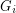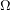# Seymour's self-minor conjecture

 Importance: High ✭✭✭
 Author(s): Seymour, Paul D.
 Subject: Graph Theory » Infinite Graphs
 Keywords: infinite graph minor
 Posted by: mdevos on: May 22nd, 2007
Conjecture   Every infinite graph is a proper minor of itself.

Robertson and Seymour famously proved that the set of all finite graphs is well-quasi-ordered by the minor relation. More precisely, if we letbe an infinite sequence of graphs, then there existso thatis a minor of. Their theory also gives us a rough structure theorem for the family of graphs without a fixed minor - a powerful tool for studying minors in finite graphs.

On the other hand, there are still large gaps in our understanding of minors of infinite graphs. For instance, while it is known that Wagner's conjecture does not hold in general for infinite graphs [T], it is possible that countably infinite graphs are well quasi-ordered.

The conjecture highlighted above is especially interesting, because (if true) it would imply the well quasi-ordering of finite graphs. Indeed, the well quasi-ordering of finite graphs is equivalent to the statement that every infinite setof finite graphs contains two distinct members, one of which is a minor of the other. This latter statement follows from the above conjecture, since we may form a single infinite graphfrom the disjoint union of the graphs in, and the proper self-minor ofgives us a pair of graphs inwith one a minor of the other.

## Bibliography

[T] R. Thomas, A counterexample to "Wagner's conjecture" for infinite graphs. Math. Proc. Cambridge Philos. Soc. 103 (1988), no. 1, 55--57. MathSciNet

* indicates original appearance(s) of problem.

### Counterexample to Seymour's self-minor conjecture

This conjecture has been shown to be false:

B. Oporowski, 'A counterexample to Seymour's self-minor conjecture.' Journal of Graph Theory, (14) 5 (521--524)

### Real Problem

The real problem, of course, is to prove Seymour's Conjectre for countable graphs (which suffices for the Graph Minor Theorem). Note that Oporowski's example involves uncountable many vertices.